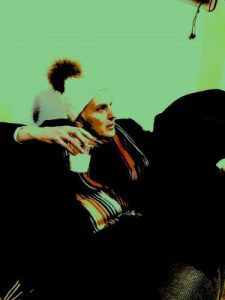Interview by Richard Marshall.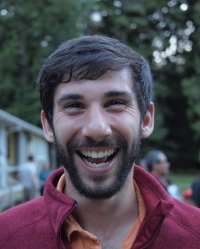Benjamin H Feintzeig focuses on the conceptual and mathematical foundations of physics, and the broader impacts of philosophy of physics on general issues in philosophy of science including interpreting physical theories, scientific representation, and scientific explanation. His primary focus is on the foundations of our best quantum theories and the classical theories that we “quantize” to arrive at them. He also has interests in philosophy of probability, epistemology, metaphilosophy, and the history of science. Here he discusses Quantization, Hilbert space conservative and Algebraic Imperialists, Bohm's interpretation, the ontological models framework, what Bell’s theorem and classical probability spaces are, why these alternative probability theories fail for quantum mechanics, the role of algebra in quantum theory, how we get out of quantum physics the actual stuff we seem to have, continuity between classical and quantum physics, how to use infinite idealizations, Nagel’s treatment of reduction, geometry, and whether we can make sense of saying physics is fundamental.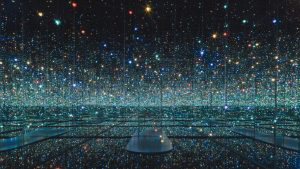3:AM: What made you become a philosopher?

Benjamin H Feintzeig:I was always interested in studying physics, but when I started taking physicsclasses in college I became dissatisfied. I would always try to ask foundational questions, which would usually result in me getting told to "shut up and calculate". I was happy to calculate, but I didn't want to shut up. So I was drawn to philosophy instead, where folks don't shy away from the difficult questions.

These days, my research is really on the border of philosophy, mathematics, and physics---I've had both philosophers and mathematicians accuse me of doing mathematics. But I couldn't be happier to be in a philosophy department, where I get exposed to many interesting topics outside of the technical complications of the sciences, and where I can keep an atmosphere of engaged discussion and collaboration in the classroom when I teach.

3:AM: Quantum mechanics is a key interest for you. Now, to construct a quantum theory you have to do a ‘quantization’. Can you give us an idea what this procedure is so that we can get a feel for what is going on and what philosophical issues arise?

BF:Quantization is the process where a physicist looks at some system they want to model and first writes down a description of that system in terms of classical physics---the physics that came before quantum mechanics, think Newton---and then somehow transforms that theory to make it "suitably quantum". The idea is that we first figure out what the relevant variables would be to describe a system classically, like the position and velocity of a rolling ball. But classical physics always allows these variables to simultaneously take on determinate values; I can always figure out where the ball is and how fast its moving. According to quantum mechanics, on the other hand, we should not simultaneously be able to know a system's position and velocity (some say system's don't even simultaneously possess determi nate position and velocity)---this is the so-called Heisenberg uncertainty principle. So we need to change our classical theory, (the classical theory is the one that defines the position and velocity variables in the first place), to accommodate the uncertainty principle by making some quantities incompatible with each other. The philosophical issue here is just that there's no consensus about what we need to change in our classical theory to make it quantum, or how we should go about implementing this change in the mathematical formalism of the theory.

There's at least some agreement about how to perform this kind of quantization procedure if we're dealing with the simplest possible system---say, a single particle like an electron moving around with no forces acting on it. In this case, we implement Heisenberg's uncertainty principle by representing the possible states of the particle using so-called "wavefunctions", which are mathematical objects corresponding to waves spread out in space. The idea here is that the particle doesn't simultaneously have a determinate position and velocity because the wave is never determinately both at a single location and moving with a single velocity.

3:AM:And can you also sketch out what is a Hilbert space, a Hilbert space Conservative and an Algebraic Imperialist?

BF:Like I said before, there's no consensus as to what mathematical formalism we should use for a quantum theory when we perform a quantization procedure. The Hilbert Space Conservative and Algebraic Imperialist advocate, roughly, for two different collections of mathematical tools for approaching quantization. A Hilbert space is the collection of all of the possible wavefunctions for an ordinary quantum mechanical system. A Hilbert Space Conservative is then an advocate for using a Hilbert space to represent the possible states of a quantum mechanical system. This is all well and good for the simple quantum mechanics systems (finite collections of non-relativistic particles moving unconstrained), but it gets more complicated for more complex systems. For example, in quantum field theory (the theory that is supposed to combine quantum mechanics and relativity), we find occasion to use states that appear to be wavefunctions in different Hilbert spaces (e.g., to describe phenomena such as symmetry breaking, free and interacting particles, distinct vacuum states). A Hilbert Space Conservative wants to just pick a single one of these Hilbert spaces and use it to construct a quantum theory, but sometimes in quantum field theory we don't want to just focus on one Hilbert space. In this case, we can use C*-algebras to represent the physically possible states and quantities of a system; a C*-algebra is a different kind of mathematical tool that gives us the freedom to talk about many different Hilbert spaces at once, in a kind of unified mathematical object. An Algebraic Imperialist is someone who advocates the use of C*-algebras to represent quantum systems in this way.

3:AM:So you’ve looked at the relationship between physical quantities and physical states and defended the Algebraic Imperialists haven’t you? This sounds epic. What’s this about, why were the Imperialists under pressure, and how do you get them out of their jam?

BF:It's true that I've provided a defense of Algebraic Imperialism against some objections. But I myself am not an Imperialist, and I'm not sure if anybody actually is. My quibble (see also my answer to 14) is just that I don't want to take on the concomitant views about physical possibility and what it means to interpret a physical theory that other authors (e.g., Aristidis Arageorgis, Laura Ruetsche) have baked into that view. Still, I do think it's promising to use algebraic tools (C*-algebras and related tools called W*-algebras) to clarify and make progress on foundational problems in quantum theories.

Even this kind of weaker view on the use of algebraic tools came under attack along with full-fledged Algebraic Imperialism though. Other mathematical physicists and philosophers argued that there are certain physical quantities that can't be modeled by a relevant C*-algebra; these quantities include "temperature", "net magnetization of a magnet", and "number of particles in a system". It would be a real problem if we couldn't talk about these quantities in our physical theory, because, for example, we need to be able to use temperature to explain phase transitions.

My defense of algebraic tools came by showing that these objectors hadn't paid enough attention to other relevant C*-algebras out there. All of these objectors focused on very particular C*-algebras that didn't have access to these important quantities, and this didn't seem to me to be inherent to the Algebraic Imperialist view. I showed (based on well known mathematical facts about these algebras) that there's another kind of algebra, called a W*-algebra, that can do the trick. A W*-algebra is a C*-algebra with a few other nice properties, and if you start with any C*-algebra there will always be some bigger W*-algebra that contains everything in the original C*-algebra. I showed that if you look at even the smallest W*-algebra containing the C*-algebra that the objectors to Algebraic Imperialism wanted to work with, then you do have enough mathematical tools for modeling all physical quantities you could ever want---including temperature, magnetization, and particle number. Since this W*-algebra is generated from the original C*-algebra using methods that I think would be acceptable to the Algebraic Imperialist, I think it saves the Algebraic Imperialist, or more generally the advocate of algebraic tools, from the objectors.

Currently, I'm working to show that algebraic tools are useful for all sorts of other interpretational purposes beyond just countering these objections.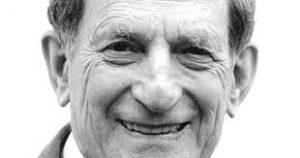3:AM:David Bohm gave an interpretation of quantum mechanics in terms of hidden variables and you’ve looked to see whether the ‘ontological models framework’ works or not. So first of all, can you sketch for us the salient things about Bohm’s interpretation, and in doing so explain why physicists are in the business of interpretation?

BF:Bohm's interpretation came in response to one of the greatest foundational problems for quantum mechanics: the infamous "measurement problem". The problem is that the ordinary formulation of quantum mechanics in terms of wavefunctions predicts that in most cases our measurements shouldn't yield any determinate result at all. The theory predicts that the process of sending a system like an electron through a measuring device will most of the time just put the measuring device into a wave-like state, where it doesn't read out a single determinate value (just like the electron doesn't have a single determinate position). But in all of our observations of electrons, we've found that when we send them through measuring devices we always get some determinate outcome. So quantum mechanics, in a sense, fails to recover the most basic feature of our experience: that measurements have results.

Bohm set out to solve this problem by providing an alternative theory to quantum mechanics. This sort of thing is sometimes called an interpretation because it keeps many of the features of quantum mechanics and then adds more, but lots of folks would say that Bohm's interpretation is really a totally new physical theory. Bohm kept the wavefunctions of ordinary quantum mechanics, but assumed in addition that all particles have determinate locations. You can imagine a physical particle then as a marble floating in the wave that gets pushed around as the wave moves. The marble is always determinately somewhere, but it moves as if it is being acted on by strange forces, and it's often hard to get the marble to go where you want it to! This theory is supposed to solve the measurement problem by asserting that all measurements are really ultimately measurements of the position of something on the measuring device (a pointer on a display or meter, for example). Even if the measuring device is associated with a spread out wavefunction, Bohm's theory tells us that the pointer on the device will always have some determinate position, just like the marble. And that's how Bohm explained the fact that measurements have outcomes.

3:AM:Ok, so what is the ‘ontological models framework’?

BF:The ontological models framework is a mathematical formalism invented to facilitate comparisons between different interpretations of quantum mechanics, or competing quantum theories. Bohm's theory isn't the only interpretation of quantum mechanics. For example, there are "spontaneous collapse" theories that assert that quantum systems will sometimes instantaneously shift from states in which their wavefunction is spread out to states in which their wavefunction is almost fully contained at some determinate position. The goal of such theories is to write down a mathematical law that predicts when these random collapses occur. The idea behind spontaneous collapse theories is that they can also solve the measurement problem by showing that after we send a quantum system like an electron through a measuring device, it's highly likely that the pointer on the measuring device collapses to a state where it at least almost has a determinate position. The ontological models framework is supposed to provide general enough mathematical tools that it can capture both Bohm's theory and the other interpretations like these collapse theories, and many more. Researchers have tended to use the ontological models framework to ask questions about, for example, the epistemic or ontological status of the wavefunction in any of these competing interpretations.

3:AM:You say this framework is a bit ambiguous and when made precise it can’t accommodate the Bohmian interpretation . Can you say something about this – and why don’t you conclude that Bohm is wrong rather than the framework?

BF:The ontological models framework has allowed researchers to prove a number of general results, including a recent one known as the PBR theorem, from which they draw sweeping philosophical or interpretational conclusions. I got worried that these theorems and their conclusions might not be as widely applicable as others hoped, so I decided to check to see whether prominent interpretations like Bohmian mechanics can be made to fit in the framework. This question turned out to be trickier to answer than I thought because there are a number of different ways of making precise what the ontological models framework is, but I found that on every way I could think of Bohmian mechanics doesn't fit. I don't think we should conclude from this that Bohm is wrong because Bohm's theory is perfectly precise and coherent on its own terms, when written down in the mathematical language Bohm used. The problem only comes when you try to shoehorn Bohmian mechanics into a mathematical language that's not as natural for it. The point I wanted to make about the ontological models framework was really rather small; I was hoping that someone would notice the ambiguities and find a new way of formulating the ontological models framework that makes it precise and allows it to accommodate Bohmian mechanics. And someone did---in my opinion, Matt Leifer has accomplished this in his review article on ontological models.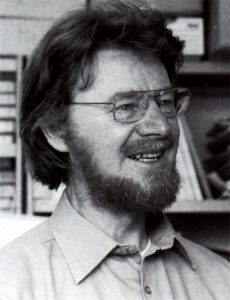3:AM: JS Bell is called the greatest philosopher of physicsin the second half of the twentieth century by Tim Maudlin(Einstein being the greatest in the first half according to Maudlin). Do you agree with this assessment

BF:I'd prefer not to comment on whether Bell is the "greatest philosopher of physics in the second half of the twentieth century". There's no doubt he made a number of major contributions to our thinking about quantum mechanics. I just worry pretty generally about picking "the greats" out of history because it tends to ignore the contributions of all but a few and contribute to the marginalization of underrepresented groups in both philosophy and the sciences. For example, I think Mary Hesse contributed a number of insights to philosophy of physics in the second half of the twentieth century that aren't given nearly enough attention. But this shouldn't be taken to undermine Bell's achievements at all.

3:AM:Can you say what Bell’s theorem and classical probability spaces are, as they feature in your thinking regarding hidden variables and incompatible observables don’t they?

We've already mentioned one famous hidden variable theory for quantum mechanics---Bohm's theory. According to Bohm's theory, the hidden variable is the determinate position of a quantum particle as it is pushed around the wavefunction. We don't usually have access to this determinate position, which is why it's called a hidden variable even though you might think it's not that hidden. But Bohm's theory is kind of weird in that it is nonlocal, meaning that stuff happening over here can affect what's happening over there, even when here and there are so far apart that not even a light signal is fast enough to get between them in the required amount of time. Bell knew about Bohm's hidden variable theory and he was interested in whether we could come up with another one that was local, meaning it prohibits these correlations between far distant events. Bell was able to show that it's not possible to come up with a hidden variable theory that reproduces the empirical predictions of quantum mechanics if we assume that the hidden variable theory satisfies a few assumptions, locality being one of them. So in a sense, Bell's theorem showed that it's not possible to come up with a hidden variable theory that is better than Bohmian mechanics, at least with regard to locality.

Bell proved his theorem first in the 1960's, but in the 1980's we gained a better understanding of it through the work of Arthur Fine and Itamar Pitowsky, among others. Fine and Pitowsky showed that the assumptions Bell made to prove his result were equivalent to assuming that we use classical probability theory to describe our hidden variables. Classical probability theory is just the ordinary theory of probability used to describe gambling with dice and cards or any of your favorite statistical analyses used throughout the natural and social sciences. So Bell's theorem shows, in a sense, that you can't come up with a hidden variable theory that reproduces the predictions of quantum mechanics if you assume the hidden variable theory obeys the rules of classical probability theory. This is important because the Fine/Pitowsky results gave some researchers motivation to claim that classical probability theory is problematic.

3:AM:What’s the idea behind the attempt to show a sense in which generalised probability spaces can serve as hidden variable theories for quantum mechanics,

BF:Fine provided a nice interpretation of his result connecting Bell's assumptions to classical probability theory, and this led to a new proposal concerning hidden variables and incompatible observables. Fine noticed that the rules of classical probability theory imply that whenever I assign probabilities to two events A and B---say, an electron exhibiting a certain position upon measurement and exhibiting a certain momentum upon measurement---I must also assign some probability to the conjunction A&B of those two events---the electron exhibiting both the position and momentum simultaneously. Classical probability theory doesn't tell us precisely what number to assign to the probability of the conjunction A&B, but it forces us to assign it some probability or other. According to Fine, this goes against the principles of quantum mechanics, which tell us some observables (like position and momentum) are incompatible. As I mentioned before, the Heisenberg uncertainty principle tells us that an electron never exhibits both a position and a momentum at the same time. So Fine said that it doesn't make sense to assign any probability at all to the conjunction A&B, when the events A and B correspond to the outcomes of incompatible observables like position and momentum, even though quantum mechanics does assign probabilities to the individual events A and B separately.

To really implement this proposal involves altering the rules of classical probability theory. There are many ways you can change the rules, and all of them go by different names, but roughly this is what generalized or alternative probability theories refer to. For example, some say we should allow for negative numbers as probabilities, while others say we should allow for imaginary numbers, and even others suggest we should change the rules for how probabilities add together. One way to change the rules of probability that I think stays closest to Fine's idea is to write down rules that allow us to not assign probabilities to some events at all---these events being the conjunctions of measurements of incompatible observables like position and momentum.

3:AM:Why do you say it fails, and what conclusions do you draw from this failure?

BF:To see why these alternative probability theories fail for quantum mechanics, it will be helpful to take a closer look at how Bell proved his theorem. He came up with a specific example of a quantum mechanical system that we can actually construct and do experiments on, but whose empirical results can't be modeled by a local hidden variable theory satisfying Bell's assumptions. This system (the EPR-Bohm setup) consists in two electrons, or two photons, produced at a common source in what's called the "singlet state" and sent to detectors very far apart where each particle is then measured. Bell showed that local hidden variable theories can't account for the predictions of quantum mechanics by providing just one concrete system whose predictions can't be captured in this way. The alternative probability theories I described above can all account for the empirical predictions of quantum mechanics for this one experiment; that's why alternative probability theories first drew attention. But just because they can account for the predictions of one hard case, that doesn't mean they can account for the predictions of all quantum systems.

There are other quantum systems that are difficult to model using hidden variable theories, besides just the two electron experiment. Around the same time Bell proved his theorem, Simon Kochen and Ernst Specker found another such system involving spin-1 particles. They proved, using slightly different assumptions than Bell, that the empirical predictions quantum mechanics makes for this system also can't be recovered by any hidden variable theory. I decided (really at the suggestion of the all-wise David Malament) to check to see whether alternative probability theories could be used to come up with a a hidden variable theory for Kochen-Specker type systems, since alternative probability theories were mostly designed to account for only the systems Bell had looked at. I was able to show, using some assumptions that I thought were pretty weak, that almost all of the alternative probability theories I considered can't account for the predictions quantum mechanics makes for these Kochen-Specker type systems. The negative probability theories, imaginary probability theories, and the probability theories that change how probabilities add all fail when tested against the Kochen-Specker systems. The conclusion here is just that either quantum mechanics can't be replaced by a hidden variable theory, even using alternative probability theories, or else some of the assumptions I made must be rejected.

3:AM: What’s the role of algebra in quantum theory?

BF:Algebraic tools have been widely applicable in both quantum and classical physics. The algebraic tools that I've been most interested in for quantum physics give us the resources to represent systems that obey something like the Heisenberg uncertainty principle. So one way of thinking about it is that algebraic tools allow us to conceive of new possibilities beyond the classical realm---in particular, they allow us to construct mathematical models that have incompatible observables and quantum superpositions. These things can also be accomplished in the traditional Hilbert space formalism of quantum mechanics, but dealing with algebras abstractly gives one slightly more freedom. For example, in quantum field theory one wants to model systems that have distinct vacuum states, but there cannot be more than one vacuum state in any given Hilbert space. Algebraic tools, however, allow us to represent even these distinct vacuum states for quantum systems.

3:AM: It’s a puzzle how we get out of quantum physics the actual stuff we seem to have, like, for example, the arrow of time or interactions between the instruments of experiments and the entities of the theories which seem almost like weird non-causal mathematical abstracts and which don’t privilege a direction of time. Am I right that this is a problem and if so, how do you think we should go about sorting it?

BF:I think you're absolutely correct that this is a problem. (I'm not sure how I feel about the arrow of time, but I think the sentiment is correct.) Quantum mechanics should be able to explain not just the results of highly esoteric experiments involving electrons and photons and particle collisions, but also why the ordinary world around us appears the way it is and why, for example, our macroscopic measuring devices actually yield results. I like to think about this as a constraint on the construction of quantum theories. We know that the old classical physics was able to give us a pretty good rough picture of how macroscopic objects like cannonballs and measuring devices behave when they're thrown about. So any quantum successor to classical physics had better be able to explain why classical physics was and is so successful, even if it's not completely correct. The idea is that even if we're living in a fully quantum world, if we cross our eyes a bit, it should still look vaguely classical. One way to accomplish this is to start from quantum physics and look at the "classical limit" of the theory. This can be understood as looking at what happens if you zoom out from microscopic quantum systems and look at larger and larger scales until they behave approximately according to the laws of classical physics. Or alternatively, if you probed a quantum system with a series of measuring devices that were less and less precise, or had larger and larger error bounds for their results, then the results of your measurements should seem to approximately obey the laws of classical physics, within reasonable bounds. This is still an ongoing project (undertaken also by mathematical physicists including Klaas Landsman), but I think it's a promising way to understand how classical behavior can arise in a quantum world.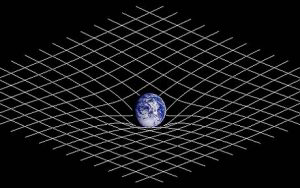3:AM: Should we require continuity between classical and quantum physics?

BF:I think so. This is the main question of much of my current research right now. I think that there already is continuity between classical and at least non-relativistic quantum mechanics, and that when we construct future quantum theories we should aim to have the same kind of continuity. Moreover, I think there is good reason to require this kind of continuity. Whenever we construct new scientific theories, we require that whatever they end up saying about the world, they should at least be able to explain why our previous scientific theories were successful, even though they've turned out to not give the whole story. When constructing quantum theories, this means that our quantum theory ought to be able to explain why classical physics was successful. Roughly, to get this kind of explanation, one shows that quantum systems behave approximately like classical systems in certain regimes (e.g., if they're big enough). The regimes where quantum systems behave like classical systems are known as the classical limit of quantum mechanics. To even construct mathematically this classical limit, or to ensure that it gives rise to the right classical behavior, one needs to require some kind of continuity between classical and quantum physics. Exactly how much classical and quantum physics need to have in common is still up for debate, but my working hypothesis is that we should require more continuity than most folks have paid attention to.

3:AM: What’s at stake when we ask whether you can define features of or deduce the behaviour of an infinite idealized system from a theory describing only finite systems?

BF:We often invoke infinite idealizations in physics even to explain rather mundane physical phenomena. For example, to model phase transitions in quantum statistical mechanics, it is standard practice to model your substance as being composed of an infinite number of particles. One prominent example of a phase transition is the transition in a magnetic material from an unmagnetized to a spontaneously magnetized phase. All of the actual magnets we observe in the world are finite in extent, but to explain their properties we need to model them as infinite systems. This should initially be puzzling, but there's an obvious response: the infinite model should be understood as an approximation to the finite real world systems, and so can still have explanatory power even while not being representationally accurate. Another way to put this in philosophical parlance is that all of our infinite models should in some sense "reduce" to finite models.

This way of thinking about how infinite idealizations are used in physics has been challenged recently by a number of philosophers of science who have argued that infinite idealization are essential for explaining, e.g., the properties of magnetic materials, and that these infinite models do not reduce to finite models. To make any headway on this question, we need to say a bit more precisely what it means for one model or theory to reduce to another. A classic philosophical treatment of reduction due to Ernst Nagel puts forth the idea that one theory reduces to another just in case all of the terms of the first theory are definable from the terms of the second theory and all of the statements of the first theory are deducible from the second theory. In this case, the first theory is sometimes called the higher-level or reduced theory, while the second theory is sometimes called the lower-level or reducing theory. If one could show that the features of our infinitely idealized models are really completely determined in this way by finite models, that would help us understand a sense in which they can be thought of as approximating real world finite systems.

3:AM:How do you handle this question, and what conclusions do you draw – and what’s the importance of these conclusions?

BF:I think that Nagel's treatment of reduction, although influential, should at least for some purposes be updated in light of modern developments of mathematics. Often physical theories are not formulated as sets of statements, so we can't apply Nagel's criteria to determine whether one theory is definable and deducible from another. Fortunately, we have modern tools from the branch of mathematics known as category theory that we can use to compare different models or different theories, and which I think shed some light on reduction. Instead of asking the question, "Is a higher-level theory completely determined by a lower-level theory by being definable and deducible from it?", category theory allows us to ask the question, "Does the higher-level theory have more or less structure than the lower-level theory?" I think this question can stand in place of the original question about reduction: if a higher-level theory has more structure than a lower-level theory, it is not reducible, but if the higher level theory has less structure than a lower-level theory, it might be reducible. Using the tools of category theory, I've been able to show that in some cases infinite models contain less structure than corresponding finite models, and so could be understood to be determined by the finite models in a natural sense. Although this doesn't show that infinite models do reduce to finite models, it at least clears away some of the roadblocks other philosophers have put forward for such a view.

3:AM: Is geometry the best mathematical representation of what quantum physics is telling us about reality?

BF:That depends on what exactly is meant by "geometry". Mathematically, algebra and geometry are considered "dual" to each other. This means, roughly, that to many algebraic notions there corresponds a geometric notion and vice versa. In fact, some aspects of the study of algebras have led to a field now called "non-commutative geometry", which is in many ways related to quantum theory. So everything previously I've said about algebraic tools can also be transferred over to geometric tools, if one has a wide enough conception of geometry.

However, it's not clear to me that any particular way of modeling quantum systems mathematically is better at representing "what quantum physics is telling us about reality". Some ways of modeling quantum systems have enough tools to capture relevant physical features, and some of those ways are even equivalent, but I'm not sure one can say much more than that. There are, of course, some mathematical tools that just won't cut it because they don't have enough resources to be able to represent quantum systems. But if we were really able to develop enough tools to model all the quantum systems we'd like to model, that would be great regardless of whether we used an algebraic or geometric formalism. I think the current problem is that we just don't yet have enough tools in any of these formalisms to construct many of the quantum theories we would like for particle physics. That's why the Clay Institute for Mathematics offers a million dollar Millennium Prize for anyone who can construct in a mathematically rigorous way the quantum theories underlying the standard model of particle physics and prove they have certain properties.

3:AM: Physics is often talked about as the most fundamental of the sciences because whatever there is is supposedly made out of stuff explained by physics. Do you think this is a good way of thinking about what physics is doing? If you do, could you flesh out the thought, and if not, what alternative would you propose is a better approach?

BF:Frankly, I get a little wary when I see the word "fundamental" because I'm often not sure what it means. I certainly don't think, for example, that we should stop doing science besides physics, or that we should force other scientists to learn and start from physics. I tend to think that scientists in all different disciplines are just doing the best they can to develop tools they can use to describe and explain (and sometimes even predict and control) the world around them. Often we hope, or even require, that the tools other scientists use be somehow compatible with the tools that physicists use, but often researchers in different disciplines are using very different approaches to very different questions. So I guess I'd like to stay agnostic on this question---after all, in physics it's sometimes easier (at least for me) to get a grasp on our own mathematical tools for representing the world than the "stuff" those tools are being used to represent.

3:AM: And finally, for the readers here at 3:AM, are there five books you can recommend that will take us further into your philosophical world?

BF:Sure, here are a few that appeal to my peculiar tastes...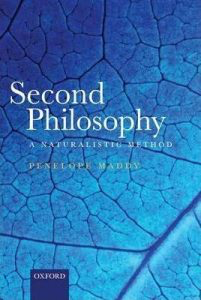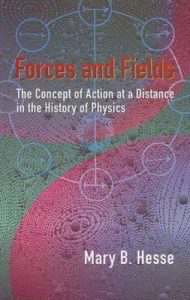Forces and Fieldsby Mary Hesse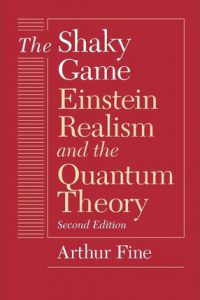The Shaky Gameby Arthur Fine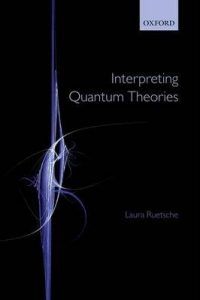Interpreting Quantum Theoriesby Laura Ruetsche

Foundations of Quantum Theoryby Klaas Landsman (well worth the read, but not for the faint of heart)